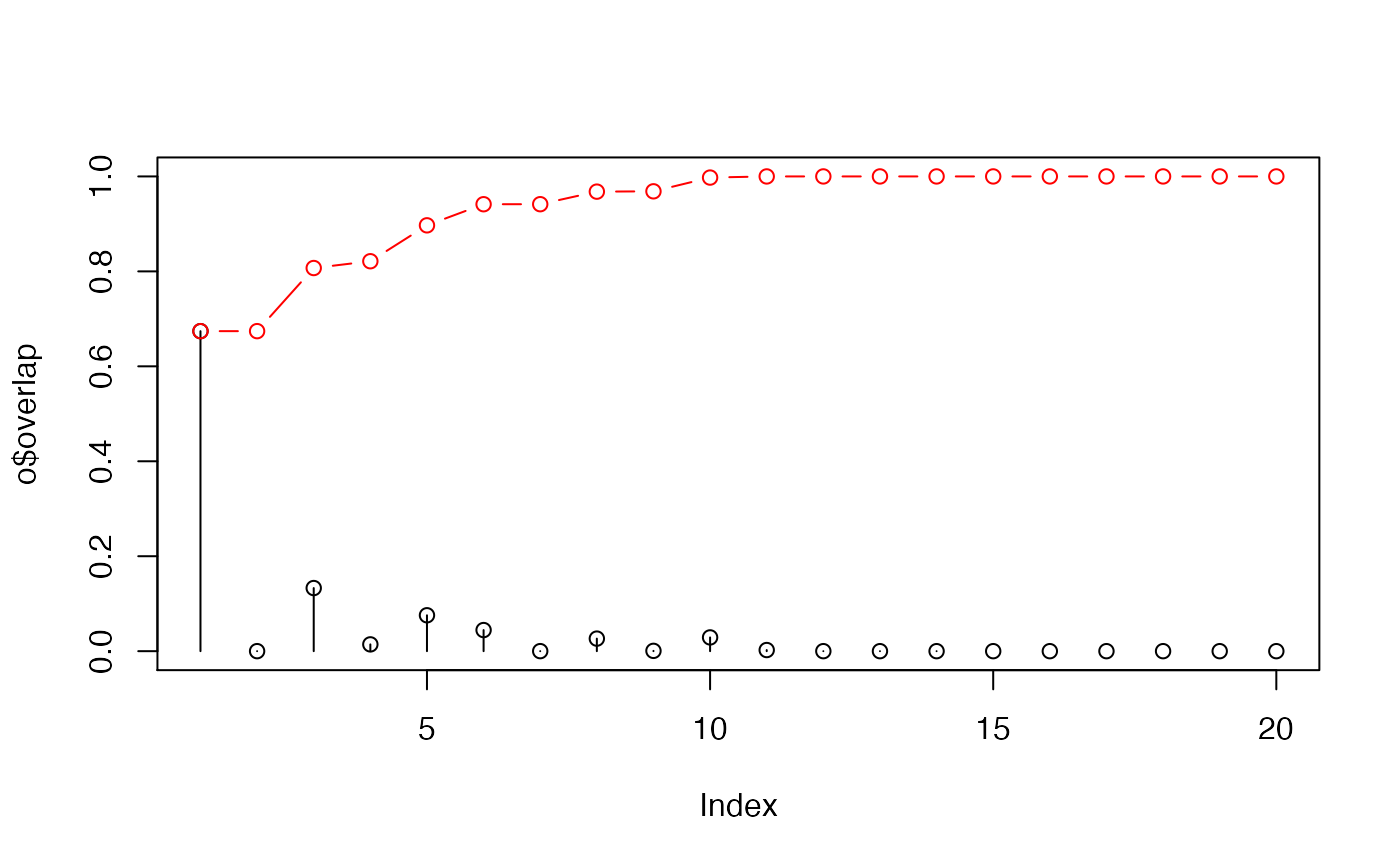Calculate the squared overlap between sets of vectors.

overlap(modes, dv, nmodes=20)

## Arguments

modes an object of class "pca" or "nma" as obtained from function pca.xyz or nma. Alternatively a 3NxM matrix of eigenvectors can be provided. a displacement vector of length 3N. the number of modes in which the calculation should be based.

## Details

Squared overlap (or dot product) is used to measure the similiarity between a displacement vector (e.g. a difference vector between two conformational states) and mode vectors obtained from principal component or normal modes analysis.

By definition the cumulative sum of the overlap values equals to one.

Structure modes$U (or alternatively, the 3NxM matrix of eigenvectors) should be of same length (3N) as dv. ## Value Returns a list with the following components: overlap a numeric vector of the squared dot products (overlap values) between the (normalized) vector (dv) and each mode in mode. overlap.cum a numeric vector of the cumulative squared overlap values. Skjaerven, L. et al. (2011) Proteins 79, 232--243. Grant, B.J. et al. (2006) Bioinformatics 22, 2695--2696. ## Author Lars Skjaerven ## See also rmsip, pca.xyz, nma, difference.vector ## Examples attach(kinesin) # Ignore gap containing positions ##gaps.res <- gap.inspect(pdbs$ali)
gaps.pos <- gap.inspect(pdbs$xyz) #-- Do PCA pc.xray <- pca.xyz(pdbs$xyz[, gaps.pos$f.inds]) # Define a difference vector between two structural states diff.inds <- c(grep("d1v8ka", pdbs$id),
grep("d1goja", pdbs$id)) dv <- difference.vector( pdbs$xyz[diff.inds,], gaps.pos$f.inds ) # Calculate the squared overlap between the PCs and the difference vector o <- overlap(pc.xray, dv) o <- overlap(pc.xray$U, dv)

# Plot results
plot(o$overlap, type='h', ylim=c(0,1)) points(o$overlap)
lines(o$overlap.cum, type='b', col='red')detach(kinesin) if (FALSE) { ## Calculate overlap from NMA pdb.a <- read.pdb("1cmk") pdb.b <- read.pdb("3dnd") ## Fetch CA coordinates sele.a <- atom.select(pdb.a, chain='E', resno=c(15:350), elety='CA') sele.b <- atom.select(pdb.b, chain='A', resno=c(1:350), elety='CA') xyz <- rbind(pdb.a$xyz[sele.a$xyz], pdb.b$xyz[sele.b\$xyz])

## Superimpose
xyz[2,] <- fit.xyz(xyz[1,], xyz[2,], 1:ncol(xyz))

## The difference between the two conformations
dv <- difference.vector( xyz )

## Calculate normal modes
modes <- nma(pdb.a, inds=sele.a)

# Calculate the squared overlap between the normal modes
# and the difference vector
o <- overlap(modes, dv)
}